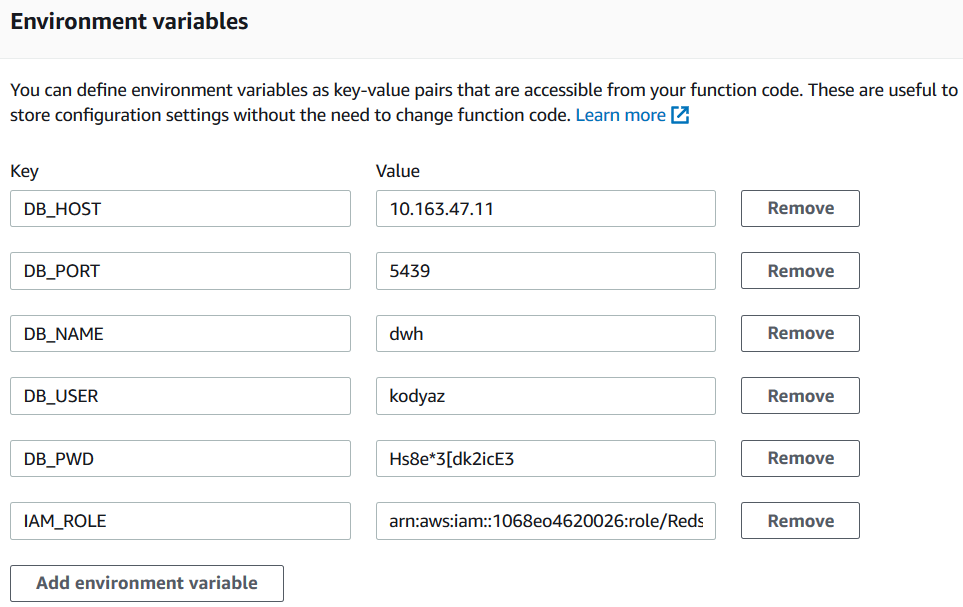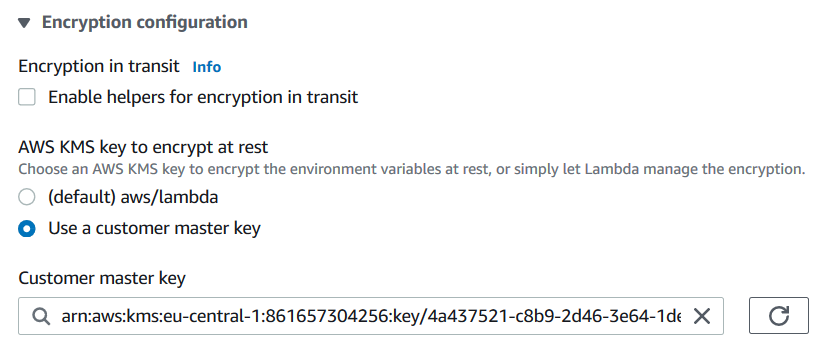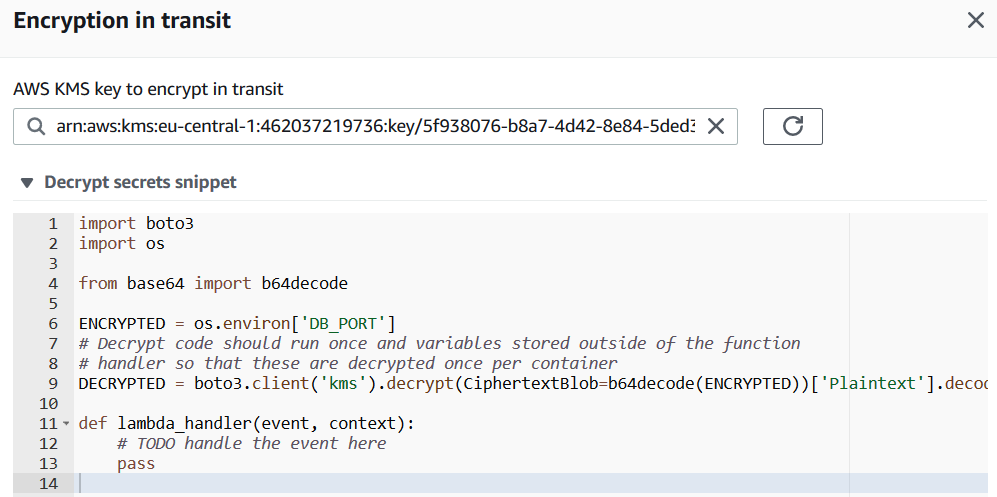Amazon Web Services AWS Tutorials and Guides Development resources, articles, tutorials, code samples and tools and downloads for ASP.Net, SQL Server, R Script, Windows, Windows Phone, AWS, SAP HANA and ABAP, like SAP UI5, Screen Personas, etc.
 Home Articles News IT Jobs Tools Sample Chapters Trainers Blogs Forums Photos Files

# Encrypt and Decrypt AWS Lambda Function Environment Variables using Python

In this AWS Lambda tutorial, I will show Python developers how they can use environment variables in their Python 3.7 Lambda function codes and how they can encrypt and decrypt these parameter values easily.

When you create a new AWS Lambda function, below the inline code editor, you will see the "Environment variables" section. Using environment variables, developers can easily create parameters that can be customized at metadata layer of a Lambda function instead of modifying its cource code. Especially if you are using the same Lambda function for different cases where only some parameters change, these environment variables are very handy. For example, for reading files from different Amazon S3 buckets, maybe the S3 bucket name can be defined as an environment variable, etc.Let's start now defining new environment variables to use as parameters in Python 3.7 source codes of our sample Lambda function.

For this tutorial, I am giving related code blocks from an AWS Lambda function which connects to an Amazon Redshift cluster database and executes some SQL commands on the target Redshift database. So I define the connection parameters like Redshift server host or IP address, database name, user credentials used to create a valid database connection, etc. as environment variables in this tutorial's sample Lambda function.

## Environment Variables in AWS Lambda Function

In this section I want to show how Lambda developers can create environment variables, assign values to these variables and encryt and decrypt the environment variables within Python code of our sample Lambda function.Press the link button "Encryption configuration" to encrypt Lambda environment variables at rest or in transit which can be used as parameters within Python code.For this AWS Lambda tutorial, I select the option of encryption at rest with default "aws/lambda" AWS KMS key instead of using a customer KMS key.

Mark the checkbox at "Encryption in transit" section in front of "Enable helpers for encryption in transit" text. This option will change the table structure of the environment variables. You will see "Encrypt" action buttons on the list where you have defined the parameters.Click on "Decrypt" button one by one.
Choose the AWS KMS key which you prefer to use for encryption Lambda function environment variable in transit.Python developers building the Lambda function code will get the code block required to decrypt each environment variable. Copy these codes we will use them in our Python code for Lambda.

``` import boto3 import os from base64 import b64decode ENCRYPTED = os.environ['DB_PORT'] # Decrypt code should run once and variables stored outside of the function # handler so that these are decrypted once per container DECRYPTED = boto3.client('kms').decrypt(CiphertextBlob=b64decode(ENCRYPTED))['Plaintext'].decode('utf-8') def lambda_handler(event, context): # TODO handle the event here ```

When you are done, press Encrypt button.

After you complete your encryption configuration for environment parameters, click Save button to return to AWS Lambda function screen.

Let's now modify you AWS Lambda function to read environment variables, decrypt them and store in string variables to use later within Lambda function Python codes

``` import json from base64 import b64decode import os import boto3 # Decrypt code should run once and variables stored outside of the function # handler so that these are decrypted once per container ENCRYPTED_DB_HOST = os.environ['DB_HOST'] DB_HOST = boto3.client('kms').decrypt(CiphertextBlob=b64decode(ENCRYPTED_DB_HOST))['Plaintext'].decode('utf-8') ENCRYPTED_DB_PORT = os.environ['DB_PORT'] DB_PORT = boto3.client('kms').decrypt(CiphertextBlob=b64decode(ENCRYPTED_DB_PORT))['Plaintext'].decode('utf-8') ENCRYPTED_DB_NAME = os.environ['DB_NAME'] DB_NAME = boto3.client('kms').decrypt(CiphertextBlob=b64decode(ENCRYPTED_DB_NAME))['Plaintext'].decode('utf-8') ENCRYPTED_DB_USER = os.environ['DB_USER'] DB_USER = boto3.client('kms').decrypt(CiphertextBlob=b64decode(ENCRYPTED_DB_USER))['Plaintext'].decode('utf-8') ENCRYPTED_DB_PWD = os.environ['DB_HOST'] DB_PWD = boto3.client('kms').decrypt(CiphertextBlob=b64decode(ENCRYPTED_DB_PWD))['Plaintext'].decode('utf-8') ENCRYPTED_IAM_ROLE = os.environ['IAM_ROLE'] IAM_ROLE = boto3.client('kms').decrypt(CiphertextBlob=b64decode(ENCRYPTED_IAM_ROLE))['Plaintext'].decode('utf-8') def lambda_handler(event, context):     print("DB Host is: " + DB_HOST)     print("DB Port is: " + DB_PORT)     print("DB Name is: " + DB_NAME)     print("DB User is: " + DB_USER)     print("DB User Password is: " + DB_PWD)     print("IAM Role is: " + IAM_ROLE) ```

After you copy above Python code on a new AWS Lambda function using Python 3.7 and execute the function after saving the codes, in Execution Result tab, you will see the string values of each corresponding environment variable.

## Troubleshooting AWS Lambda Function Code for Environment Variables

Please note if the Lambda function execution fails to succeed, please take the differences between Python 2.7 and Python 3.7. This AWS Lambda function is created using Python 3.7

A possible error is caused by code copied from Python 2.7 Lambda functions.
For example if you get following error: Syntax error in module 'lambda_function': Missing parentheses in call to 'print'.
Then you are using the "print" command without parentheses

``` # wrong print "DB_HOST: " + DB_HOST # correct print("DB Host is: " + DB_HOST) ```

Again if the Python developer has copied the environment variable decryption code from a Lambda function built using Python 2.7, following error message can be faced where the decrypted value is being used like "print" commands, etc.

TypeError: can only concatenate str (not "bytes") to str

This error is caused because in Python 3.7 to get the decrypted paramter value as string, it has to be decoded using .decode('utf-8') in addition to its version in Python 2.7

``` # wrong: valid in Python 2.7 but not in Python 3.7 DB_HOST = boto3.client('kms').decrypt(CiphertextBlob=b64decode(ENCRYPTED_DB_HOST))['Plaintext'] # works successfully with Python 3.7 DB_HOST = boto3.client('kms').decrypt(CiphertextBlob=b64decode(ENCRYPTED_DB_HOST))['Plaintext'].decode('utf-8') ```

One last error case, if you experience following error message: name 'b64decode' is not defined then it is possible that you forgot to import the b64decode.
from base64 import b64decode , to the beginning section where you import modules into your Lambda function.

Similar to above error: "name 'os' is not defined" error is again related with missing Python module within imports sections. Just add "import os"
To resolve "name 'boto3' is not defined" error, add import boto3 code line

AWS Tutorials

AWS Tutorials

AWS